Courses

# Competition Level Test: Solution Of Triangles- 1

## 30 Questions MCQ Test Mathematics For JEE | Competition Level Test: Solution Of Triangles- 1

Description
This mock test of Competition Level Test: Solution Of Triangles- 1 for JEE helps you for every JEE entrance exam. This contains 30 Multiple Choice Questions for JEE Competition Level Test: Solution Of Triangles- 1 (mcq) to study with solutions a complete question bank. The solved questions answers in this Competition Level Test: Solution Of Triangles- 1 quiz give you a good mix of easy questions and tough questions. JEE students definitely take this Competition Level Test: Solution Of Triangles- 1 exercise for a better result in the exam. You can find other Competition Level Test: Solution Of Triangles- 1 extra questions, long questions & short questions for JEE on EduRev as well by searching above.
QUESTION: 1

Solution:
QUESTION: 2

Solution:
QUESTION: 3

### In a ΔABC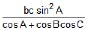is equal to

Solution:
QUESTION: 4

In a triangle ABC, (a + b + c) (b + c – a) = kbc, if

Solution:
QUESTION: 5

If in a triangle ABC, right angle at B, s – a = 3 and s – c = 2, then

Solution: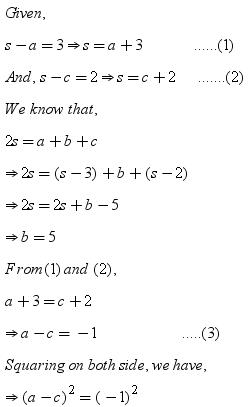QUESTION: 6

In a ΔABC if b + c = 3a, then cot B/2 . cot C/2 has the value equal to

Solution:
QUESTION: 7

In a ΔABC, A = 2π/3, b - c = 3√3 cm and ar(ΔABC) = 9√3/2 cm2. Then a is

Solution:

BD is drawn perpendicular to side AC in the given triangle ABC.
Now, in triangle ABD, BD = c sinA = √3 c/2
Area of triangle ABC = BD*AC/2 = √3 bc/4
also, it is given that area of triangle is 9 root 3/2 cm2.
therefore, bc = 18
Since,  cosA = (b2 + c2 - a2)/2bc
-1/2 = {(b-c)2 + 2bc -a2}/2bc
-1/2 = (27 + 36 - a2)/18
a2 = 81
a = 9

QUESTION: 8

If in a ΔABC, Δ = a2 – (b – c)2, then tan A =

Solution:

Given, Δ=a2−(b−c)2
Now as we know that s=(a+b+c)/2
or, a=2s−(b+c)
putting this value of a in Δ=a2−(b+c)2, we get
Δ=[2s−(b+c)]2−(b−c)2
Δ=[4s2+(b+c)2−4s(b+c)]−(b−c)2
Δ=4s2−4s(b+c)+[(b+c)−(b−c)2]
Δ=4s2−4s(b+c)+4bc
Δ=4s2−4sb−4sc+4bc
Δ=4s(s−b)−4c(s−b)
Δ=(4s−4c)(s−b)
Δ=4(s−c)(s−b)
1/4= (s-b)(s−c)/Δ ...............(1)
Now as we know that tanA/2 = {((s−b)(s−c)/[s(s-a)]}1/2
multiply both side by ((s−b)(s−c))1/2
(s−b)(s−c) =tanA/2[s(s-a)(s-b)(s-c)]1/2
(s−b)(s−c)/Δ= tanA/2................(2)
by using 1 and 2
1/4= tanA/2
tanA = 2tanA/2/(1-tan2A/2)
= [2(1/4)]/[1-(1/4)2]= 8/15

QUESTION: 9

If R denotes circumradius, then in ΔABC,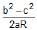is equal to

Solution: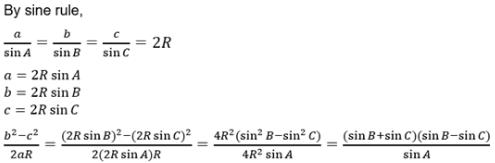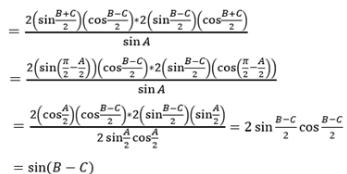QUESTION: 10

If a ΔABC, if b = 2 cm, c = √3 and ∠A = π/6, then values of R is equal to

Solution:
QUESTION: 11

In a ΔABC, the value of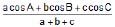is equal to

Solution:
QUESTION: 12

If the sides of a triangle are 3 : 7 : 8, then R : r is equal to

Solution:
QUESTION: 13

In a right angled triangle R is equal to

Solution:
QUESTION: 14

If the area of triangle is 100 sq. cm, r1 = 10 cm and r2 = 50 cm, then the value of (b – a) is equal to

Solution:
QUESTION: 15

In a ΔABC, the inradius and three exradii are r, r1, r2 and r3 respectively. In usual notations the value of r . r1 . r2 . r3 is equal to

Solution:
QUESTION: 16

In a ΔABC if r1 > r2 > r3, then

Solution:
QUESTION: 17

The product of the arithmetic mean of the lengths of the sides of a triangle and harmonic mean of the lengths of the altitudes of the triangle is equal to

Solution:
QUESTION: 18

In a ΔABC, if AB = 5 cm, BC = 13 cm and CA = 12 cm, then the distance of vertex A from the side BC is (in cm)

Solution: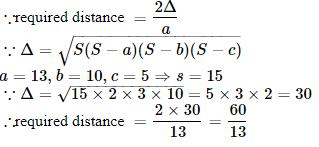QUESTION: 19

If AD, BE and CF are the medians of ΔABC, then (AD2 + BE2 + CF2) : (BC2 + CA2 + AB2) is equal to

Solution:
QUESTION: 20

In a triangle ABC, right angled at B, the inradius is

Solution:
QUESTION: 21

If H is the orthocentre of a triangle ABC, then the radii of the circle circumscribing the triangles BHC, CHA and AHB are respectively equal to

Solution:
QUESTION: 22

The distance between the middle point of BC and the foot of the perpendicular form A is

Solution:
QUESTION: 23

Let f, g, h be the lengths of the perpendiculars from the circumcentre of the ΔABC on the sides a, b and c respectively. If a/f + b/g + c/h = l (abc/fgh) then the value of l is

Solution:
QUESTION: 24

In a triangle ABC, if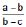=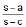, then r1, r2, r3 are in

Solution:
QUESTION: 25

A triangle is inscribed in a circle. The vertices of the triangle divide the circle into three arcs of length 3, 4 and 5 units. Then area of the triangle is equal to

Solution:
QUESTION: 26

If in a triangle ABC, the line joining the circumcentre and incentre is parallel to BC, then cos B + cos C is equal to

Solution:
QUESTION: 27

If the incircle of the ΔABC touches its sides respectively at L, M and N and if x, y, z be the circumradii of the triangles MIN, NIL and LIM where I is the incentre then the product xyz is equal to

Solution:
QUESTION: 28

If in a ΔABC, = , then the value of tan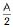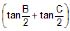is equal to

Solution:
QUESTION: 29

If in a ΔABC, ∠A = , then tan C/2 is equal to

Solution:
QUESTION: 30

In a acute angled triangle ABC, AP is the altitude. Circle drawn with AP as its diameter cuts the sides AB and AC at D and E respectively, then length DE is equal to

Solution: